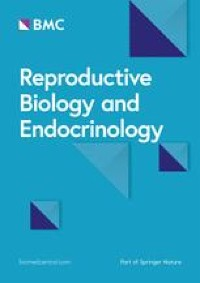# Betaine attenuate chronic restraint stress-induced changes in testicular damage and oxidative stress in male mice – Reproductive Biology and Endocrinology

#### ByXingqi Meng, Lixuan Peng, Jie Xu, Dongming Guo, Wenyu Cao, Yang Xu and Suyun Li

May 21, 2022### Effects of betaine on organ and sperm parameters in chronic stressed mice

On the 3rd week, the animals were injected intraperitoneally with betaine at 200 mg/Kg (Fig. 1A). One-way ANOVA showed a significant difference among the groups in the sperm count (F(2,15) = 18.42, P < 0.0001), viability rate (F(2,15) = 19.85, P < 0.0001) and sperm deformity rate (F(2,15) = 7.824, P = 0.00047) (Table 4). Bonferroni post-test analysis revealed that the sperm count (t = 6.058, P < 0.0001) and viability rate (t = 6.254, P < 0.0001) decreased significantly in the CRS + NS group compared with the Control + NS group, while the sperm deformity rate (t = 1.845, P = 0.2548) significantly increased in the CRS + NS group. BET treatment can reverse this situation (sperm count: t = 2.719, P = 0.0475; viability rate: t = 3.796, P = 0.0053; deformity rate: t = 1.845, P = 0.2548).

### Betaine treatment improves the adverse effect of chronic restraint stress on the structure of testis in mice

The H&E results showed that the testis of CRS + NS group appeared obvious pathological structural changes, including significantly reduced diameter of the seminiferous tubules of the testes and loosely arranged spermatogenic cells, which was restored after the injection of BET. And the results were further verified by Johnsen’s scores, one-way ANOVA suggested that there were significantly differences among the groups in the Johnsen’s scores (F(2,51) = 63.94, P < 0.0001). Bonferroni post-test analysis revealed that the Johnsen’s scores of CRS + NS group were significantly drop compared with the Control + NS group (t = 11.10, P < 0.0001), which was reversed by BET treatment (t = 7.403, P < 0.0001) (Fig. 2).

### Effect of betaine on spermatogenic cells in the testis of chronic stressed mice

To further investigate the effects of betaine on various spermatogenic cells in the testes of CRS mice, the levels of Androgen receptor (AR,a marker of Sertoli cells), CD29 (a marker of spermatogonial stem cells) and INSL3 (a marker of Leydig cells) were detected by PCR (Fig. 3A). As shown in Fig. 3, there were significant differences among the three groups in the expression of spermatogenic cell markers (AR:F(2,13) = 12.71, P = 0.0009; CD29:F(2,13) = 8.301, P = 0.0048; INSL3:F(2,15) = 81.94, P = 0.0001). Bonferroni post-test analysis revealed that the level of spermatogenic cell marker was significantly reduced in the CRS + NS group compared with Control + NS group (AR:t = 4.961, P = 0.0008; CD29:t = 4.070, P = 0.0040; INSL3:t = 4.289, P = 0.0019), which was reversed by BET treatment (AR:t = 3.355, P = 0.0155; CD29:t = 1.999, P = 0.2009; INSL3:t = 12.59, P < 0.0001).

And the results were further verified by western blot (Fig. 3B-C). There were significant differences among the three groups (AR:F(2,23) = 1.976, P = 0.1615; CD29:F(2,23) = 10.45, P = 0.0006; GFRA1:F(2,15) = 16.03, P = 0.0002). Bonferroni post-test analysis revealed that the levels of spermatogenic cell marker was significantly reduced in the CRS + NS group compared with Control + NS group (AR:t = 1.984, P = 0.1780; CD29:t = 4.493, P = 0.0005; GFRA1:t = 5.440, P = 0.0002), which was recovered by BET treatment (AR:t = 1.157, P = 0.7773; CD29:t = 3.095, P = 0.0153; GFRA1:t = 1.361, P = 0.5811).

The results of immunohistochemistry showed that there were significant differences among the three groups in the expression of INSL3 (Fig. 3D) (F(2, 76) = 14.12, P < 0.0001). Bonferroni post-test analysis revealed that The levels of INSL3 was significantly reduced in the CRS + NS group compared with Control + NS group (t = 4.506, P < 0.0001), which were recovered by BET treatment (t = 4.788, P < 0.0001).

Taken together, these results indicated that BET treatment led to increased expression of various spermatogenic cell in the testis of CRS mice.

### Effects of betaine on testicular apoptosis and serum testosterone levels in chronically stressed mice

Since inflammation can induce apoptosis in testicular cells, we also used TUNEL apoptosis detection kit to detect the apoptosis level of testicular cells in mice testis. As shown in Fig. 4A-C, there were significant differences among the three groups in the Apoptotic tubule index (F(2,15) = 10.63, P = 0.0013) and apoptotic cell index (F(2,15) = 27.38, P < 0.0001). Bonferroni post-test analysis revealed that the apoptosis levels of Apoptotic tubule index (t = 4.362, P = 0.0017) and apoptotic cell index (t = 7.355, P < 0.0001) were significantly increased in the CRS + NS group compared with Control + NS group, which was reversed by BET treatment (Apoptotic tubule index:t = 3.477, P = 0.0101; Apoptotic cell index:t = 4.385, P = 0.0016).

To understand the effect of BET on serum testosterone levels in mice, we used a testosterone (T) enzyme-linked immunosorbent assay kit to detect serum testosterone levels in peripheral blood of mice. As shown in Fig. 4F, there were significant differences among the three groups in serum testosterone levels in mice. (F(2,23) = 6.990, P = 0.0042). Bonferroni post-test analysis revealed that serum testosterone levels was significantly reduced in the CRS + NS group compared with Control + NS group (t = 3.668, P = 0.0038), but not reversed by BET treatment (t = 1.288, P = 0.6317).

Thus, BET treatment can reduce the apoptosis level of testicular cells in the testis of CRS mice.

### Betaine reduces testicular oxidative stress in chronic stressed mice

We measured testis oxidative Glutathione (GSSG) content, Reduced Glutathione (GSH) content and malondialdehyde (MDA) content (Fig. 5). As shown in Fig. 5, there were significant differences among the three groups in the levels of GSSG (F(2,15) = 29.25, P < 0.0001), GSH (F(2,15) = 60.39, P < 0.0001), MDA (F(2,33) = 71.17, P < 0.0001). Bonferroni post-test analysis revealed that the levels of MDA (t = 8.134, P < 0.0001) and GSSG (t = 7.012, P < 0.0001) were significantly increased in the CRS + NS group compared with Control + NS group, which were reversed by BET treatment (MDA:t = 11.63, P < 0.0001; GSSG:t = 6.151, P < 0.0001). Bonferroni post-test analysis revealed that the level of GSH was significantly reduced in the CRS + NS group compared with Control + NS group (t = 6.034, P < 0.0001), which was revserd by BET treatment (t = 10.97, P < 0.0001). From the results we know that BET can prevent the over-oxidative stress in testis of CRS mice.

### Effects of betaine on the level of inflammatory cytokines in the testis of chronic stressed mice

As peroxidative stress can cause inflammation in the testis, we also used PCR to detect the expression of inflammatory factors in the testis of mice. As shown in Fig. 6, significant differences was found amoung the three groups in the levels of IL-1 β(F(2,11) = 12.49, P = 0.0015), IL-6(F(2,13) = 20.46, P < 0.0001) and TNF-α(F(2,12) = 10.37, P = 0.0024). Bonferroni post-test analysis revealed that the levels of inflammatory markers were significantly increased in the CRS + NS group compared with Control + NS group (IL-1β:t = 3.823, P = 0.0085; IL-6:t = 6.297, P < 0.0001; TNF-α:t = 2.587, P = 0.0713), which was reversed by BET treatment (IL-6:t = 3.823, P = 0.0063; TNF-α:t = 4.536, P = 0.0020) except IL-1β (t = 0.6004, P > 0.9999).

These results showed that BET treatment could prevent CRS-induced increased inflammatory cytokines in the testis.